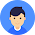# What is javascript operators

JavaScript operators are symbols that are used to perform operations on operands. For example:

1. var sum=10+20;

Here, + is the arithmetic operator and = is the assignment operator.

There are following types of operators in JavaScript.

1. Arithmetic Operators

2. Comparison (Relational) Operators

3. Bitwise Operators

4. Logical Operators

5. Assignment Operators

6. Special Operators

## JavaScript Arithmetic Operators

Arithmetic operators are used to perform arithmetic operations on the operands. The following operators are known as JavaScript arithmetic operators.

OperatorDescriptionExample
-Subtraction20-10 = 10
*Multiplication10*20 = 200
/Division20/10 = 2
%Modulus (Remainder)20%10 = 0
++Incrementvar a=10; a++; Now a = 11
--Decrementvar a=10; a--; Now a = 9

## JavaScript Comparison Operators

The JavaScript comparison operator compares the two operands. The comparison operators are as follows:

OperatorDescriptionExample
==Is equal to10==20 = false
===Identical (equal and of same type)10==20 = false
!=Not equal to10!=20 = true
!==Not Identical20!==20 = false
>Greater than20>10 = true
>=Greater than or equal to20>=10 = true
<Less than20<10 = false
<=Less than or equal to20<=10 = false

## JavaScript Bitwise Operators

The bitwise operators perform bitwise operations on operands. The bitwise operators are as follows:

OperatorDescriptionExample
&Bitwise AND(10==20 & 20==33) = false
|Bitwise OR(10==20 | 20==33) = false
^Bitwise XOR(10==20 ^ 20==33) = false
~Bitwise NOT(~10) = -10
<<Bitwise Left Shift(10<<2) = 40
>>Bitwise Right Shift(10>>2) = 2
>>>Bitwise Right Shift with Zero(10>>>2) = 2

## JavaScript Logical Operators

The following operators are known as JavaScript logical operators.

OperatorDescriptionExample
&&Logical AND(10==20 && 20==33) = false
||Logical OR(10==20 || 20==33) = false
!Logical Not!(10==20) = true

## JavaScript Assignment Operators

The following operators are known as JavaScript assignment operators.

OperatorDescriptionExample
=Assign10+10 = 20
+=Add and assignvar a=10; a+=20; Now a = 30
-=Subtract and assignvar a=20; a-=10; Now a = 10
*=Multiply and assignvar a=10; a*=20; Now a = 200
/=Divide and assignvar a=10; a/=2; Now a = 5
%=Modulus and assignvar a=10; a%=2; Now a = 0

## JavaScript Special Operators

The following operators are known as JavaScript special operators.

OperatorDescription
(?:)Conditional Operator returns value based on the condition. It is like if-else.
,Comma Operator allows multiple expressions to be evaluated as single statement.
deleteDelete Operator deletes a property from the object.
inIn Operator checks if object has the given property
instanceofchecks if the object is an instance of given type
newcreates an instance (object)
typeofchecks the type of object.
voidit discards the expression's return value.
yieldchecks what is returned in a generator by the generator's iterator.

1.1.2.1.